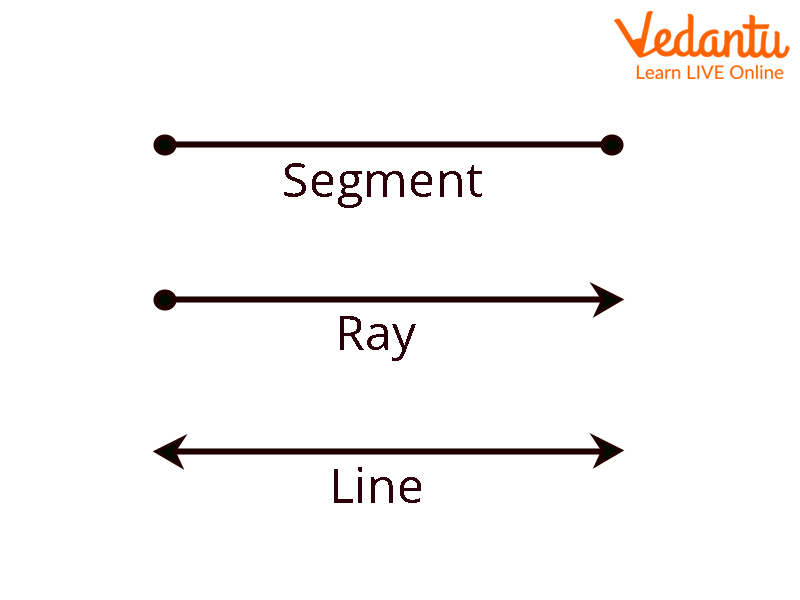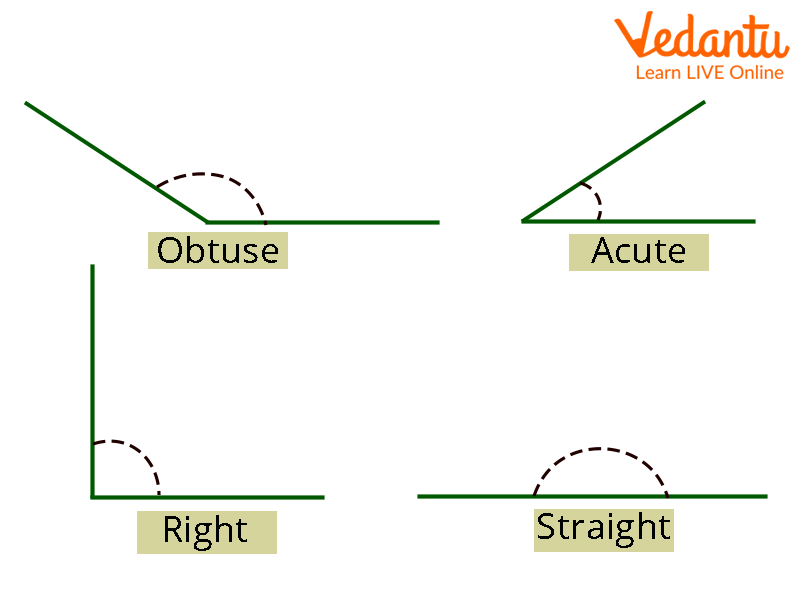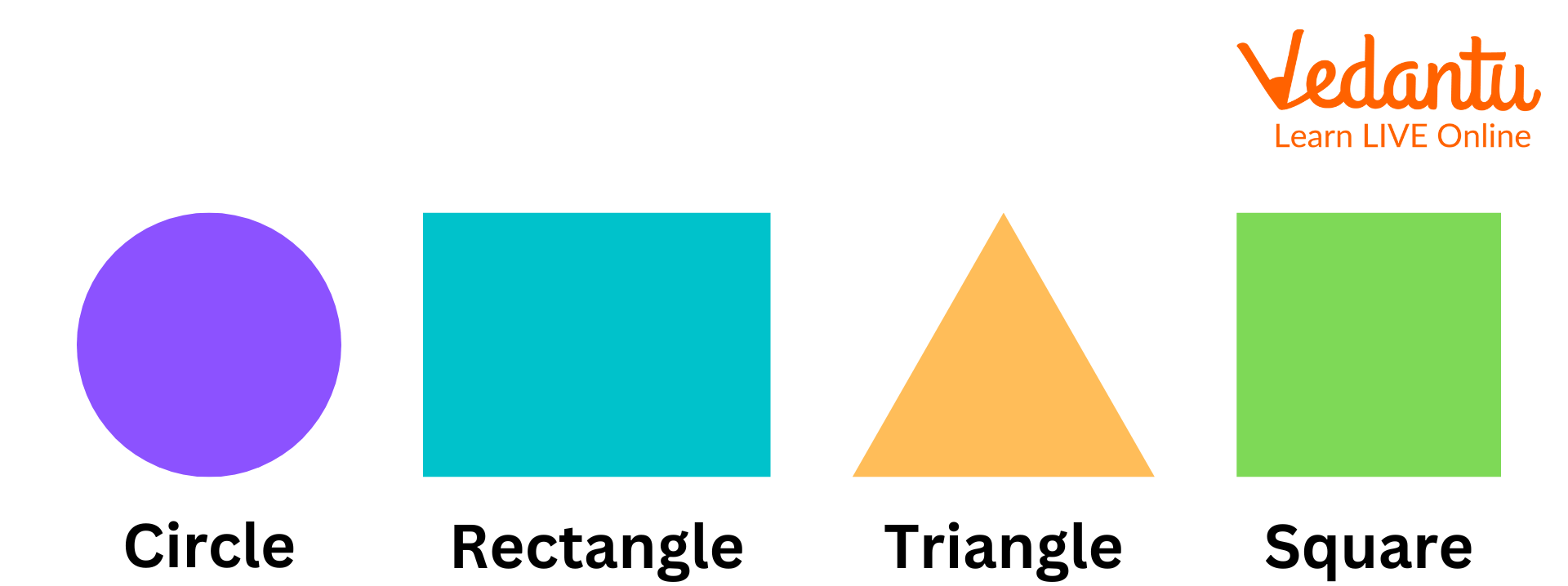Courses
Courses for Kids
Free study material
Free LIVE classes
More

# Geometry for Class 5## Introduction

2D forms like triangles, squares, rectangles, and circles are examples of flat shapes in plane geometry. Solid geometry also refers to three-dimensional forms like cubes, cuboids, etc. as solids. Fundamental geometry is built on the coordinate geometry of points, lines, and planes. The area of mathematics known as geometry is concerned with the dimensions, sizes, forms, and angles of a wide range of common objects. Geometry comes from the Ancient Greek terms "geo" and "metron," which both imply "measuring." There are two-dimensional shapes and three-dimensional forms in Euclidean geometry.

Geometry's many sorts of forms make it easier for us to comprehend the shapes we encounter every day. Geometric ideas allow us to compute the area, perimeter, and volume of forms.

## Basic Terms Used in Geometry are:

### Point

On a plane, a point is a particular area or position. Usually, a dot represents them. It's important to understand that a point is not an object, but rather a position. Remember that a point has just one location and, ideally, no dimensions.

### Line

The line has no thickness, is straight (no curves), and goes in both directions without coming to an end (infinitely). It is significant to remember that an unlimited number of points come together to form a line.

Line Segment - A line is referred to as a Line Segment if it has a starting point and an ending point.

Ray - If there is a starting point and no ending point in a line then it becomes a ray.Line segment, Ray, Line

## Class 5 Shapes and Angles

Two rays that share a termination and are collectively referred to as the angle's sides and vertices form an angle in planar geometry.

• Acute Angle - A Acute angle is a smaller angle than a straight angle, i.e. It can be in the \[0^\circ \] to \[90^\circ \].

• Obtuse Angle: Obtuse angles are those that are greater than \[90^\circ \] but less than \[180^\circ \].

• Right Angle: A right angle is a \[90^\circ \]of angle.

• Straight Angle - A straight angle is an angle created by a straight line, and it has a \[180^\circ \].Angles

## Basic Geometrical Figures and Shapes

Some basic geometrical figures are given below:

### 1. Triangle

The polygonal shape known as a triangle has three sides, three edges, and three vertices. Additionally, the total of its interior angles is \[180^\circ \].

### 2. Circle

A circle is a collection of all points that are all at the same set distance from a common central point.

### 3. Square

The quadrilateral known as the "square" has four equal sides and angles, and each of its vertices has a \[90^\circ \] angle.

### 4. Rectangle

The internal angles of a rectangle, which has four sides, are all exactly 90 degrees. At each corner or vertex, the two sides come together at a straight angle. The rectangle differs from a square because its two opposite sides are of equal length.

### 5. Polygon

A polygon is a closed, two-dimensional structure with straight line segments in mathematics. It does not have three dimensions. There aren't any curved surfaces in a polygon. A polygon must have three sides at a minimum. Only at its terminal must each side of a line segment cross another line segment.Different Geometrical Shapes

## Conclusion:

Geometry is a branch of mathematics that focuses on measuring, describing, and relating points, lines, angles, surfaces, and solids. The four fundamental components of geometry are a point, a line, an angle, and a plane. Flat forms in plane geometry include 2-dimensional shapes including triangles, squares, rectangles, and circles. Three-dimensional forms like a cube, cuboid, cone, etc. are also referred to as solids in solid geometry. The coordinate geometry of points, lines, and planes is the foundation of fundamental geometry.

## Solved Examples

1. What is the total angle of the triangle?

Ans: The Total of angles of a triangle is 180 degrees.

2. What kind of shape is a cone?

Ans: A cone is a solid, three-dimensional shape having a single vertex and a circular base. From the base to the vertex, the cone's surface narrows.

3. Give a few differences between a square and a rectangle.

Ans:

 Square Rectangle All sides are of equal length. Only the opposite sides are of equal length. Diagonals of a square are the perpendicular bisector of each other. Diagonals of a rectangle are not perpendicular bisectors of each other. Area of square = Side2 Area of Rectangle = Length x Breadth Perimeter of square = 4 x (side-length) Perimeter of rectangle = 2(Length+Breadth)

Last updated date: 31st May 2023
Total views: 46.5k
Views today: 0.42k

## FAQs on Geometry for Class 5

1. How is geometry utilized?

Different shapes and sizes, such as circles, squares, cubes, triangles, etc., are constructed and measured using geometry.

2. What applications of geometry?

In daily life, geometry is widely used. Its primary uses are in the construction industry, including the building of buildings, roads, dams, bridges, etc.

3. Why is geometry important?

Geometry is necessary for our daily life to comprehend the various shapes and quantify them using area and volume.